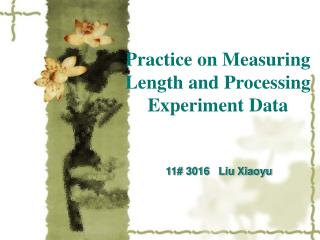DownloadDownload PresentationPractice on Measuring Length and Processing Experiment Data

Practice on Measuring Length and Processing Experiment Data

Download PresentationPractice on Measuring Length and Processing Experiment Data

- - - - - - - - - - - - - - - - - - - - - - - - - - - E N D - - - - - - - - - - - - - - - - - - - - - - - - - - -
Presentation Transcript

1. Practice on Measuring Length and Processing Experiment Data 11# 3016 Liu Xiaoyu

2. What we need to do in this experiment? To obtain the volume of a hollow cylinder and a sphere • What measuring tools we can use ? A metal ruler, a vernier caliper & a micrometer • Why we need to do such an experiment? 1. Know the correct use of the vernier caliper & the micrometer 2. Do some exercises for processing experiment data

3. 1).Metal ruler 1.Introduction of tools

4. Parameters for a ruler: Graduation value (i): defined as the value between two closed graduation marks. Instrument error ( ): defined as half of the graduation value.

5. 2). Vernier caliper

6. Parameters for a vernier caliper: Graduation value: defined as i=a/n. Instrument error: same value as the graduation value. Rules for reading: L=L0+ki

7. 3). Micrometer

8. Parameters for a micrometer: Graduation value: also can be defined as i=a/n Instrument error: half of the graduation value. Rules for reading: The final result = the reading – the original reading

9. 2. Experiment data 1) Height of the hollow cylinder (measured by the metal ruler)mm

10. 2). Outside & inside diameter of the hollow cylinder (measured by the vernier caliper) mm

11. 3.Data processing 1). Volume of the hollow cylinder

12. 2).Volume of the sphere